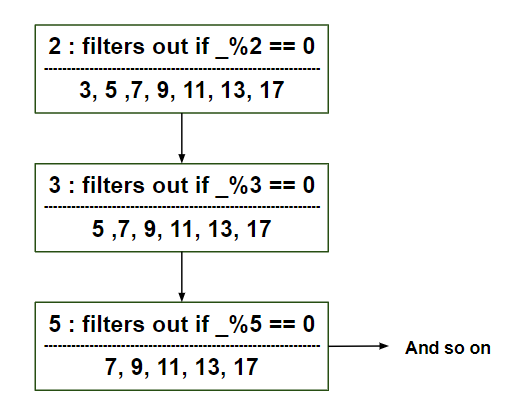# Scala | Sieve of Eratosthenes

• Last Updated : 26 Aug, 2019

Eratosthenes of Cyrene was a Greek mathematician, who discovered an amazing algorithm to find prime numbers.

Step 1 : Creating an Int Stream

 `def` `numberStream(n``:` `Int)``:` `    ``Stream[Int] ``=` `Stream.from(n)``     ` `println(numberStream(``10``))`

Output of above step is Stream(10, ?).

Step 2 : Sieve of Eratosthenes function

 `// Defining Sieve of Eratosthenes``def` `sieve``_``of``_``Eratosthenes(stream``:` `Stream[Int])``:` `    ``Stream[Int] ``=` `stream.head ``#::` `sieve``_``of``_``Eratosthenes(``        ``(stream.tail) ``        ``filter (x ``=>` `x ``%` `stream.head !``=` `0``)``        ``)``         ` `println(sieve``_``of``_``Eratosthenes(numberStream(``10``)))`

Output of above step is Stream(10, ?).

Step 3 : Extracting “N” number of primes

 `val` `no``_``of``_``primes ``=` `sieve``_``of``_``Eratosthenes(numberStream(``2``))`` ` `// Selecting number of primes``println(no``_``of``_``primes)``(no``_``of``_``primes take ``7``) foreach { ``    ``println(``_``) ``    ``}`

Output of above step is Stream(2, ?)
2
3
5
7
11
13
17
.

Below is the complete program

 `def` `numberStream(n``:` `Int)``:` `    ``Stream[Int] ``=` `Stream.from(n)``      ` `println(numberStream(``10``))`` ` `// Defining Sieve of Eratosthenes``def` `sieve``_``of``_``Eratosthenes(stream``:` `Stream[Int])``:` `    ``Stream[Int] ``=` `stream.head ``#::` `sieve``_``of``_``Eratosthenes(``        ``(stream.tail) ``        ``filter (x ``=>` `x ``%` `stream.head!``=` `0``)``        ``)``          ` `println(sieve``_``of``_``Eratosthenes(numberStream(``10``)))`` ` ` ` `val` `no``_``of``_``primes ``=` `sieve``_``of``_``Eratosthenes(numberStream(``2``))`` ` `// Selecting number of primes ``println(no``_``of``_``primes)``(no``_``of``_``primes take ``7``) foreach { ``    ``println(``_``) ``    ``}`

Output:

```Stream(10, ?)
Stream(10, ?)
Stream(2, ?)
2
3
5
7
11
13
17
```

Insights from the code

• Using stream.form(), a stream is created which is generating successive numbers. And this number starts off from the argument.
• A number stream is given to the “sieve_of_Eratosthenes” method. This method by filtering out the elements, lazily generates the successive elements.

Below is the complete working code with explanation:Working: abc() method inserts the debug statement in the filter() method. If an element is not evenly divisible by the head, the stream treats it as a good element. The code prints it and return true. Otherwise the filtered out sequence is printed and finally the stream is returned.
Some modification is done in sieve_of_Eratosthenes method so as to use the stream creation – abc() method. Elements are taken out from the recursive stream and is printed.

 `object` `Sieve ``extends` `App {``    ``def` `abc(s``:` `Stream[Int], head``:` `Int) ``=` `{ ``        ``val` `r ``=` `s filter {``            ``x ``=>` `{``                ``if` `(x ``%` `head !``=` `0``) {``                    ``println()``                    ``println(s``"\${x} is not evenly divisible by \${head}"``)``                     ` `                    ``true``                    ``} ``                ``else` `{``                    ``println()``                    ``println(s``"\${x} is evenly divisible by \${head}. So Discard \${x}"``)``                ``false``                    ``}``                ``}``            ``}``        ``r``    ``}``    ``def` `numberStream(g``:` `Int)``:` `Stream[Int] ``=` `Stream.from(g)``     ` `    ``def` `sieve``_``of``_``Eratosthenes(stream``:` `Stream[Int])``:` `    ``Stream[Int] ``=` `stream.head ``#::` `sieve``_``of``_``Eratosthenes(``        ``abc(stream.tail, stream.head))``     ` `    ``val` `no``_``of``_``primes ``=` `sieve``_``of``_``Eratosthenes(numberStream(``2``))``    ``(no``_``of``_``primes take ``7``) foreach {``        ``println(``_``)``    ``}``}`

Output :

```2

3 is not evenly divisible by 2
3

4 is evenly divisible by 2. So Discard 4

5 is not evenly divisible by 2

5 is not evenly divisible by 3
5

6 is evenly divisible by 2. So Discard 6

7 is not evenly divisible by 2

7 is not evenly divisible by 3

7 is not evenly divisible by 5
7

8 is evenly divisible by 2. So Discard 8

9 is not evenly divisible by 2

9 is evenly divisible by 3. So Discard 9

10 is evenly divisible by 2. So Discard 10

11 is not evenly divisible by 2

11 is not evenly divisible by 3

11 is not evenly divisible by 5

11 is not evenly divisible by 7
11

12 is evenly divisible by 2. So Discard 12

13 is not evenly divisible by 2

13 is not evenly divisible by 3

13 is not evenly divisible by 5

13 is not evenly divisible by 7

13 is not evenly divisible by 11
13

14 is evenly divisible by 2. So Discard 14

15 is not evenly divisible by 2

15 is evenly divisible by 3. So Discard 15

16 is evenly divisible by 2. So Discard 16

17 is not evenly divisible by 2

17 is not evenly divisible by 3

17 is not evenly divisible by 5

17 is not evenly divisible by 7

17 is not evenly divisible by 11

17 is not evenly divisible by 13
17
```

My Personal Notes arrow_drop_up